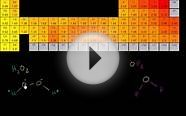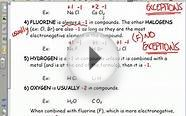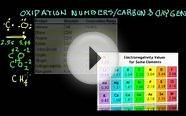# Oxidation Numbers Chart

Top

The oxidation number (or oxidation state) of an element represents the number of electrons that are gained, transferred or shared. The oxidation number of an element is a positive or negative number assigned to an element which can be used to predict the chemical bonds that it will form with other elements.

An atom has a positive oxidation number when it loses electrons. On the other hand, an atom has a negative oxidation number if electrons are gained by the atoms when it combines with other atoms.

Oxidation numbers are one of the basis of chemistry. Oxidation numbers chart is used for converting an element into an elemental state. This can be done by adding or subtracting an electron from the atom. This concept is called as the oxidation numbers chart. Oxidation numbers having the atom, which is present at the center and the compound.

An oxidation number can be positive, negative or zero. The oxidation number of every atom in a free element is 0. By "free element" we mean an element that is not combined with another element. Therefore Na, He, O2 and P4 are free elements and each of the atoms that comprise them has an oxidation number of 0.

The periodic table of the elements shows the common oxidation states of the elements. These values appear in the upper right side of each elements. Ionic substances are present in the oxidation number. For transferring an electron we are using the red-ox reaction. In the oxidation number, the reduction process and the ionic process can take place. Also the oxidation numbers can be defined as the process of removing the number of atoms to get a single atom.

The oxidation numbers are having positive charge, negative charge and the zero charge. By using the covalent process, the charges are not allowed.

### Solved Examples

Question 1: Find the oxidation number for the given molecule. CIO3
Solution:

Step 1: Take a as an oxidation number for the unknown element.

Step 2: For the first element, we are going to find the oxidation number,

a + ( -2 x 3) = 1

a – 6 = -1 (or)

x = 5

Therefore, the value 5 is the oxidation number.

Question 2: Find the oxidation number for the given molecule. BrF3